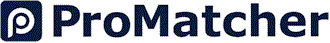| Heat Pumps ■ Promote Your Business
 Heat Pumps ProMatch Directory Cost Report

# Heat Pump Efficiency

Choosing a High-Efficiency Heat Pump

There are two separate ratings that rate heat pump efficiency. The SEER measures cooling efficiency while the HSPF measures heating efficiency.

SEER Rating

The SEER, or Seasonal Energy Efficiency Ratio, rating is a measure of cooling efficiency developed by the Air Conditioning, Heating & Refrigeration Institute. This rating represents a ratio of the heat pump's cooling output (in BTUs) divided by the total energy input over a period of time (expressed in watt-hours). Heat pumps are required to have a minimum SEER rating of 13.

Heat pumps with a higher SEER are more efficient and typically more expensive. However, as a result, the higher initial cost can be quickly offset by the monthly energy savings. An efficient heat pump will have a SEER between 14 and 18.

HSPF Rating

The Heating Seasonal Performance Factor (HSPF) is a measure of heating efficiency. A high-efficiency heat pump will have a higher HSPF rating. Although these units cost more, the cost can be offset by future energy savings.

The HSPF ratio is the heat output (in BTUs) during the heating season divided by the amount of electricity used (in watt-hours). The HSPF is similar to the SEER rating, but it also incorporates supplemental heating needs in the calculation. For example, the energy used to defrost the unit, in addition to any back-up systems, are included in the heat output calculation. Heat pumps are required to have a minimum HSPF rating of 6.8, but the most efficient systems have a HSPF of 10.

Related:

Cost of Heat Pump Installation

Geothermal Heat Pumps vs. Air-Source Heat Pumps